• GRNN_python 广义回归神经网络进行回归
• 广义回归神经网络matlab编程，根据已知数据进行训练并对数据进预测。
• 提出使用广义回归神经网络进行敦煌壁画的数字化修复保护研究。通过各向异性扩散去除.待修复壁画图像的噪声，使用形态学膨胀算子提取待修复区域的边界像素点，利用与待修复区域边界邻域.像素相似的样本像素块作为广义...
• 基于光纤应变分布与布里渊散射谱频移的关系，提出了利用广义回归神经网络提取布里渊谱的应变特征方法。将布里渊谱的频率、增益分别作为广义回归神经网络的输入矢量和目标矢量，对广义回归神经网络进行训练和仿真，...
• 使用基于广义回归神经网络的机器学习对 2 类和 3 类问题进行一维矩阵分类。 它还包含一个基于矩阵的 AND 门示例和大小为 12 和 3 的输入样本matlab
• MATLAB源码集锦-基于广义回归神经网络货运量预测代码
• 然后选取二者判别结果相同的数据作为训练和测试样本,运用广义回归神经网络,对二者判别结果分歧的钻孔数据进行二次判别。结果表明:广义回归神经网络性能良好,预测准确度高。此外,这种综合判别方法也提高了饱和砂土...
• 针对获取数据不足等特点,首次在煤炭的预测中运用动态广义回归神经网络(D-GRNN)的方法,预测了中国未来近十年内的煤炭预测值,得到了比较合理的预测值。运用预测数据对国家的煤炭宏观调控做出了合理评价。
• 基于广义回归神经网络的Bootstrap滑坡位移区间预测研究论文
•研究论文
• 【机器学习】广义回归神经网络（GRNN）的python实现一、广义回归神经网络原理1.1、GRNN与PNN的关系2.2、GRNN的网络结构二、广义回归神经网络的优点与不足2.1、优点2.2、不足三、GRNN的python实现参考资料 一、广义...
【机器学习】广义回归神经网络（GRNN）的python实现一、广义回归神经网络原理1.1、GRNN与PNN的关系2.2、GRNN的网络结构二、广义回归神经网络的优点与不足2.1、优点2.2、不足三、GRNN的python实现参考资料
一、广义回归神经网络原理
1.1、GRNN与PNN的关系
广义回归神经网络（Generalized Regression Neural Network）的网络结构类似于RBF神经网络。与概率神经网络（PNN）相同，GRNN也是一个前向传播的网络，不需要反向传播求模型参数；不同的是GRNN用于求解回归问题，而PNN用于求解分类问题。关于GRNN的数学原理可以参考资料【1】。
2.2、GRNN的网络结构
如下图所示，GRNN与PNN一样也是一个四层的网络结构。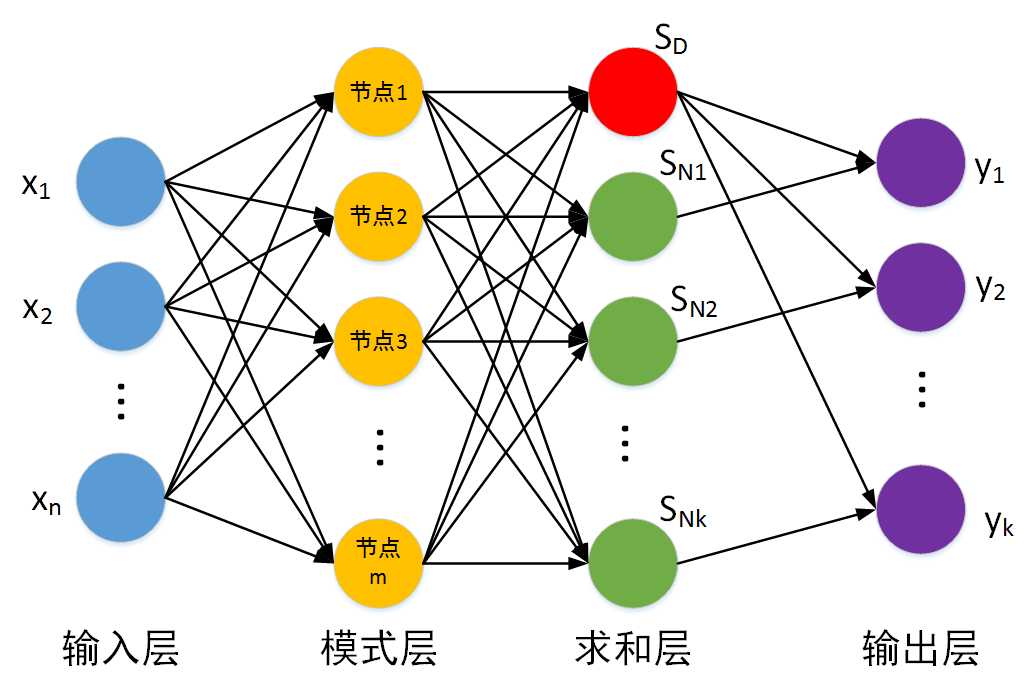对于回归问题训练数据集包括样本特征集与标签集，假设样本特征集为：$\left\{ {tr{x_1},tr{x_2}, \cdots ,tr{x_m}} \right\}$，每一个样本的维度为$n$，即  $tr{x_i} = [{x_1},{x_2}, \cdots ,{x_n}]$。标签集为：$\left\{ {tr{y_1},tr{y_2}, \cdots ,tr{y_m}} \right\}$，每一个标签的维度为$k$，即  $tr{y_i} = [{y_1},{y_2}, \cdots ,{y_k}]$。
输入层：输入测试样本，节点个数等于样本的特征维度。
模式层：（与PNN的模式层相同）计算测试样本与训练样本中的每一个样本的Gauss函数的取值，节点个数等于训练样本的个数。
第$i$个测试样本$te{x_i}$与第$j$个训练样本$trx_j$之间的Gauss函数取值（对于测试样本$x$，从第$j$个模式层节点输出的数值）为：
$Gauss(te{x_i} - tr{x_j}) = {e^{ - \frac{{\left\| {te{x_i} - tr{x_j}} \right\|}}{{2{\delta ^2}}}}}$
其中$\delta$是模型的超参数（机器学习模型中，超参数是在开始学习过程之前设置值的参数），需要提前设定，也可以通过寻优算法（GA，QGA，PSO，QPSO等）获得。
求和层：节点个数等于输出样本维度加1（$k+1$），求和层的输出分为两部分，第一个节点输出为模式层输出的算术和，其余$k$个节点的输出为模式层输出的加权和。
假设对于测试样本$tex$，模式层的输出为$\left\{ {{g_1},{g_2}, \cdots ,{g_m}} \right\}$。
求和层第一个节点的输出为：
${S_D} = \sum\limits_{i = 1}^m {{g_i}}$
其余$k$个节点的输出为：
${S_{Nj}} = \sum\limits_{i = 1}^m {{y_{ij}}{g_i},j = 1,2, \cdots ,k}$
其中加权系数$y_{ij}$为第$j$个模式层节点对应的训练样本的标签的第$j$个元素。
输出层：输出层节点个数等于标签向量的维度，每个节点的输出等于对应的求和层输出与求和层第一个节点输出相除。
二、广义回归神经网络的优点与不足
2.1、优点
收敛快：没有模型参数需要训练，收敛速度快。
非线性逼近：以径向基网络为基础，具有良好的非线性逼近性能。
2.2、不足
计算复杂度高：每个测试样本要与全部的训练样本进行计算。
空间复杂度高：因为没有模型参数，对于测试样本全部的训练样本都要参与计算，因此需要存储全部的训练样本。
三、GRNN的python实现
完整python代码与样本地址：https://github.com/shiluqiang/GRNN_python
本博文采用数据集为sine函数的数据集，将前190组数据作为训练集，后10组数据作为测试集。数据集中特征维度为1，标签维度也为1，因此GRNN的网络结构应该是（1：190：2：1）。
首先，计算模式层的输出。
def distance(X,Y):
'''计算两个样本之间的距离
'''
return np.sqrt(np.sum(np.square(X-Y),axis = 1))

def distance_mat(trainX,testX):
'''计算待测试样本与所有训练样本的欧式距离
input:trainX(mat):训练样本
testX(mat):测试样本
output:Euclidean_D(mat):测试样本与训练样本的距离矩阵
'''
m,n = np.shape(trainX)
p = np.shape(testX)
Euclidean_D = np.mat(np.zeros((p,m)))
for i in range(p):
for j in range(m):
Euclidean_D[i,j] = distance(testX[i,:],trainX[j,:])[0,0]
return Euclidean_D

def Gauss(Euclidean_D,sigma):
'''测试样本与训练样本的距离矩阵对应的Gauss矩阵
input:Euclidean_D(mat):测试样本与训练样本的距离矩阵
sigma(float):Gauss函数的标准差
output:Gauss(mat):Gauss矩阵
'''
m,n = np.shape(Euclidean_D)
Gauss = np.mat(np.zeros((m,n)))
for i in range(m):
for j in range(n):
Gauss[i,j] = math.exp(- Euclidean_D[i,j] / (2 * (sigma ** 2)))
return Gauss

然后，计算求和层输出。
def sum_layer(Gauss,trY):
'''求和层矩阵，列数等于输出向量维度+1,其中0列为每个测试样本Gauss数值之和
'''
m,l = np.shape(Gauss)
n = np.shape(trY)
sum_mat = np.mat(np.zeros((m,n+1)))
## 对所有模式层神经元输出进行算术求和
for i in range(m):
sum_mat[i,0] = np.sum(Gauss[i,:],axis = 1) ##sum_mat的第0列为每个测试样本Gauss数值之和
## 对所有模式层神经元进行加权求和
for i in range(m):
for j in range(n):
total = 0.0
for s in range(l):
total += Gauss[i,s] * trY[s,j]
sum_mat[i,j+1] = total           ##sum_mat的后面的列为每个测试样本Gauss加权之和
return sum_mat

最后，计算输出层的输出。
def output_layer(sum_mat):
'''输出层输出
input:sum_mat(mat):求和层输出矩阵
output:output_mat(mat):输出层输出矩阵
'''
m,n = np.shape(sum_mat)
output_mat = np.mat(np.zeros((m,n-1)))
for i in range(n-1):
output_mat[:,i] = sum_mat[:,i+1] / sum_mat[:,0]
return output_mat

结果展示：

测试样本标签
回归结果

0.624676
0.557845

0.816921
0.881194

1.04449
0.916176

-0.30906
-0.293338

0.267336
0.126166

1.01882
0.815953

0.409871
0.296891

0.920009
0.912512

-0.112378
-0.0923096

0.768894
0.86055

参考资料
1、https://blog.csdn.net/guoyunlei/article/details/76101899?locationNum=4&fps=1


展开全文GRNN 神经网络 RBF神经网络
• GRNN的数据预测-基于广义回归神经网络货运量预测 matlab程序供大家学习使用
• 针对总体人体下肢运动模式识别率低，提出了一种基于多源信息和广义回归神经网络（GRNN）的下肢运动模式识别方法。通过足底压力信息将人体日常下肢动作分解为不同的动作片段以组成识别目标集，选择下肢表面肌肉电信号...
• 针对回归分析问题提出了一种动态确定结果合成权重的神经网络集成构造方法，在训练出个体神经网络之后，根据各个体网络在输入空间上对训练样本的预测误差，应用广义回归网络来动态地确定各个体网络在特定输入空间上的...神经网络集成 BP网络
• 广义回归神经网络（GRNN） 广义回归神经网络是径向基神经网络的一种，GRNN具有很强的非线性映射能力和学习速度，比RBF具有更强的优势，网络最后普收敛于样本量集聚较多的优化回归，样本数据少时，预测效果很好，还...
广义回归神经网络（GRNN）
广义回归神经网络是径向基神经网络的一种，GRNN具有很强的非线性映射能力和学习速度，比RBF具有更强的优势，网络最后普收敛于样本量集聚较多的优化回归，样本数据少时，预测效果很好，还可以处理不稳定数据。虽然GRNN看起来没有径向基精准，但实际在分类和拟合上，特别是数据精准度比较差的时候有着很大的优势。
关于RBF,GRNN与PNN
RBF网络是一个两层的网络，除了输入输出层之外仅有一个隐层。隐层中的转换函数是局部响应的高斯函数，而其他前向型网络，转换函数一般都是全局响应函数。由于这样的不同，要实现同样的功能，RBF需要更多的神经元，这就是RBF网络不能取代标准前向型网络的原因。但是RBF的训练时间更短。它对函数的逼近是最优的，可以以任意精度逼近任意连续函数。隐层中的神经元越多，逼近越较精确.
径向基神经元和线性神经元可以建立广义回归神经网络，它是径RBF网络的一种变化形式，经常用于函数逼近。在某些方面比RBF网络更具优势。
径向基神经元和竞争神经元还可以组成概率神经网络。PNN也是RBF的一种变化形式，结构简单训练快捷，特别适合于模式分类问题的解决。
一、GRNN网络结构
GRNN是RBF的一种改进，结构相似。区别就在于多了一层求和层，而去掉了隐含层与输出层的权值连接（对高斯权值的最小二乘叠加）。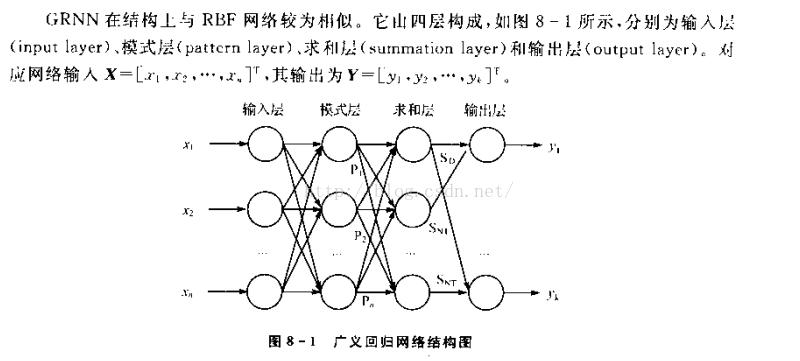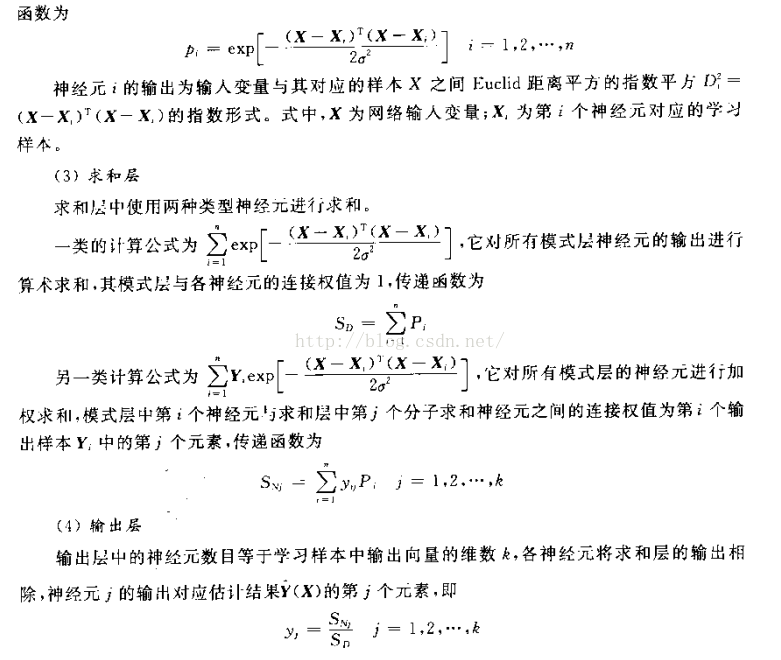文字解析：
1.输入层为向量，维度为m，样本个数为n，线性函数为传输函数。
2.隐藏层与输入层全连接，层内无连接，隐藏层神经元个数与样本个数相等，也就是n，传输函数为径向基函数。
3.加和层中有两个节点，第一个节点为每个隐含层节点的输出和，第二个节点为预期的结果与每个隐含层节点的加权和。
4.输出层输出是第二个节点除以第一个节点。
二、GRNN的MATLAB实现
%设置变量
data=-9:1:8;
x=-9:.2:8;
label=[129,-32,-118,-138,-125,-97,-55,-23,-4,2,1,-31,-72,-121,-142,-174,-155,-77];
%由于grnn没有权值的处理，不用训练使得他的处理速度很快
%隐藏层的处理
chdis=dist(x’,data)
chgdis=chgdis’;
%加和输出
y=t*chgdis./(sum(chgdis))
查看图像效果
plot(x,y,‘o’)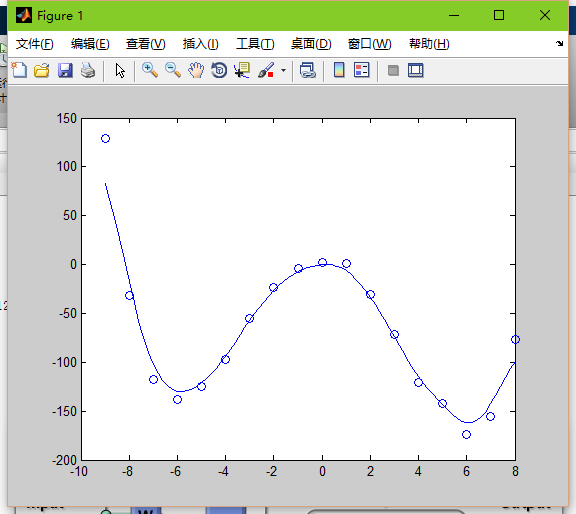展开全文• 针对采煤机故障征兆和故障的非线性对应关系,采用广义回归神经网络作为故障诊断的智能分类器。输入层为采煤机的故障特征参数,中间层为径向基神经元,感知待诊断故障向量与训练样本的相似度,输出层为故障模式分类。分析...
• 针对煤与瓦斯突出存在诸多不确定影响因素的特点,在研究煤与瓦斯突出机理和广义回归神经网络的基础上,建立了煤与瓦斯突出的广义回归神经网络预测模型,提出了广义回归神经网络中的光滑因子优化选择算法,以提高模型预测...
• 基于改进广义回归神经网络和主成分分析的宽带DOA估计研究论文
• 研究大尺度IP骨干网络流量矩阵估计，通过使用广义回归神经网络来捕捉流量矩阵特征，将流量矩阵估计描述成马氏距离下的最优化过程，能成功克服流量矩阵估计的病态特性，获得精确的估计值。仿真结果表明，该估计算法...网络流量 马氏距离
• 本资源是GRNN的数据预测-基于广义回归神经网络货运量预测的matlab仿真，GRNN具有很强的非线性映射能力和柔性网络结构以及高度的容错性和鲁棒性，适用于解决非线性问题。GRNN在逼近能力和学习速度上较RBF网络有更强的...
• 在介绍广义回归神经网络（GRNN）基本算法、网络结构及平滑参数确定方法的基础上,提出将误差序列的均方值作为网络性能的评价指标并采用最小误差对应的平滑参数,建立了GRNN的预测模型。提出了确定输入神经元数目的方法...
• 针对高阶正交幅度调制和大线宽相干光正交频分复用(CO-OFDM)系统，提出了一种基于广义回归神经网络(GRNN)的非线性均衡算法。将接收端进行相位噪声恢复之后的批量数据作为训练数据样本，通过训练学习得到GRNN的唯一...
• 提出一种基于广义回归神经网络的无参考模糊图像质量评价方法。该方法首先通过相位一致变换生成待评测图像的相位一致图像，然后利用灰度共生矩阵计算相位一致图像的信息熵、能量、对比度、相关性和同质性5个特征，...
• 以广东省番禹区沙洲和石楼镇岛地区的1657个高程点为样本点,把其分为A,B,C组各200个高程点,A+B组400个高程点,A+B+C组600个高程点作为训练数据集,在Matlab7.1和ArcGIS9.2平台上分别应用广义回归神经网络(GRNN)、普通...
• 针对现有煤泥浮选加药量预测精度不足的现状,提出了基于广义回归神经网络(GRNN)的浮选加药量预测。首先介绍了GRNN的网络结构,然后通过交叉验证搜索算法确定了模型的结构参数,最后通过与BP网络模型的比较,得出了该模型...
• 果蝇优化算法 优化 广义回归神经网络 对不常用备件进行ABC分类
• 为实现对双陷波超宽带(UWB)天线的精准神经网络建模,提出了一种利用改进的果蝇算法(FOA)优化广义回归神经网络(GRNN)的建模方法。该方法通过扩大果蝇搜索范围,在味道判定公式中引入调整项来实现果蝇算法的改进,并用...
• %% 学习目标:广义回归神经网络 %% 训练速度快 非线性映射能力强 常用于函数逼近 clear all; close all; …… …… …… （省略部分代码，完整代码可以下载） …… …… …… net=newgrnn(P,T,0.3); %径向基函数的...
%%  学习目标:广义回归神经网络
%%   训练速度快 非线性映射能力强   常用于函数逼近
clear all;
close all;
……
……
……
（省略部分代码，完整代码可以下载）
……
……
……
net=newgrnn(P,T,0.3);      %径向基函数的分布密度是0.3
y=sim(net,P);
figure;
plot(P,T,’:+’,P,T-y,’-o’);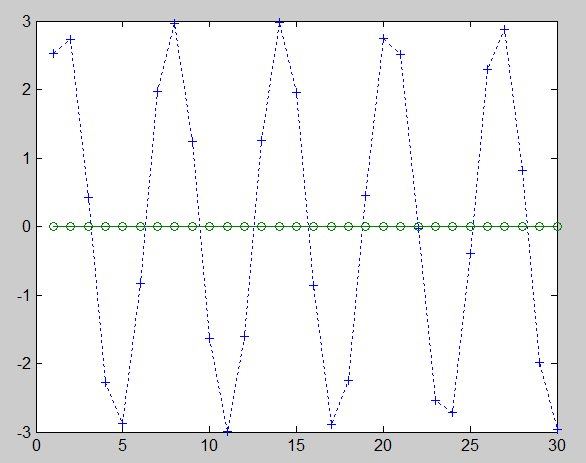(完整代码及资料下载地址)
新上线了一门视频课程——二十八天精通matlab基础编程-在线视频教程-CSDN学院  https://edu.csdn.net/course/detail/24947


展开全文matlab 神经网络...